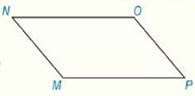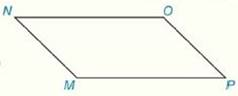Chapter 4.CR, Problem 17CRElementary Geometry For College St...

7th Edition
Alexander + 2 others
ISBN: 9781337614085

Solutions

Chapter
SectionElementary Geometry For College St...

7th Edition
Alexander + 2 others
ISBN: 9781337614085
Textbook Problem

Review Exercises Using the information from Review Exercise 16, determine which diagonal ( M O - or P N - ) would be longer.Exercise 16: Given: ▱ M N O P m ∠ M = 4 x m ∠ O = 2 x + 50 Find:   m ∠ M   and   m ∠ PTo determine

To Find:

The longest diagonal of the parallelogram MNOP.

Explanation

Properties Used:

1. Two consecutive angles of a parallelogram are supplementary.

2. The opposite angles of a parallelogram are congruent.

3. In a parallelogram with unequal pairs of consecutive angles, the longer diagonal lies opposite the obtuse angle.

Calculation:

It is given that MNOP is a parallelogram with mM=4x and mO=2x+50.

In the parallelogram MNOP, M and O are opposite angles.

By the property ‘opposite angles of a parallelogram are congruent’, mM=mO.

4x=2x+50

2x=50

x=25°

Substituting the value of x in measure of angle M,

mM=4x=425=100°

In the parallelogram MNOP, mM and mP are consecutive angles

Still sussing out bartleby?

Check out a sample textbook solution.

See a sample solution

The Solution to Your Study Problems

Bartleby provides explanations to thousands of textbook problems written by our experts, many with advanced degrees!

Get Started

39.

Mathematical Applications for the Management, Life, and Social Sciences

Fill in each blank: 3qt=pt

Elementary Technical Mathematics

True or False: f(x) = x2 is decreasing for 10 x 1.

Study Guide for Stewart's Single Variable Calculus: Early Transcendentals, 8th

In Exercises 3538, find the transpose of each matrix. 

Finite Mathematics for the Managerial, Life, and Social Sciences

True or False: is a convergent series.

Study Guide for Stewart's Multivariable Calculus, 8th